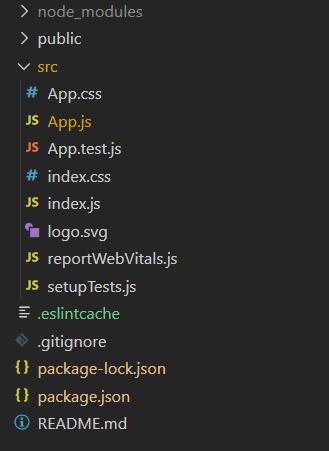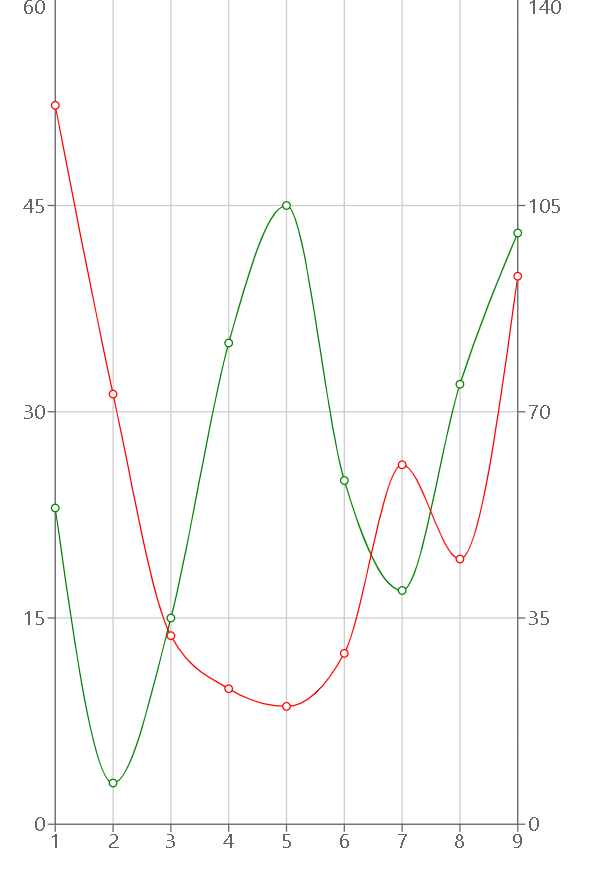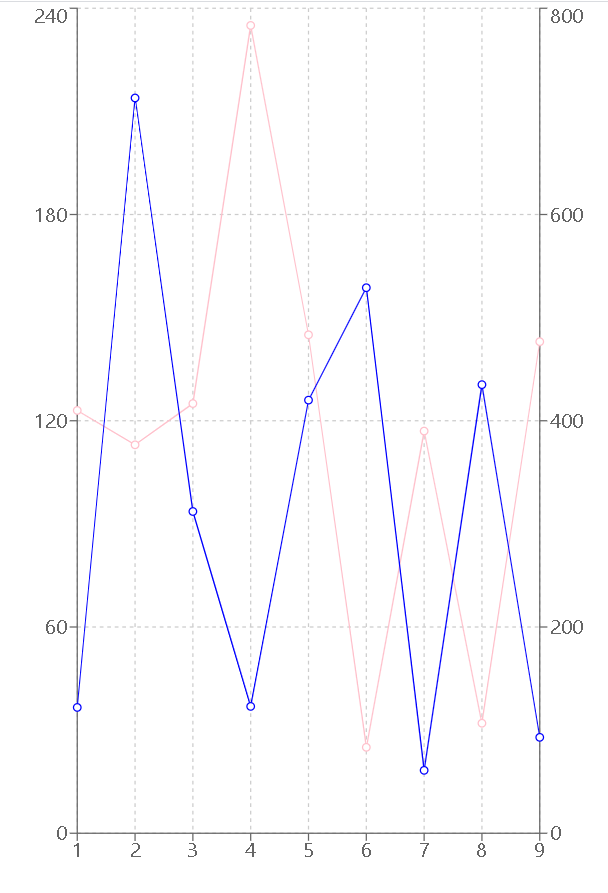Open in App
Not now

# Create a BiAxial Line Chart using Recharts in ReactJS

• Difficulty Level : Expert
• Last Updated : 01 Aug, 2021

Introduction: Rechart JS is a library that is used for creating charts for React JS. This library is used for building Line charts, Bar charts, Pie charts, etc, with the help of React and D3 (Data-Driven Documents).

BiAxial line chart is a Line chart that has two Y-Axis instead of one. It is used to compare two different series of data points with different Y-Axis.

Approach: To create a Biaxial Line chart in react using recharts, we firstly create a data variable with all the data points and labels. Then we create a cartesian grid and all three axes i.e. one X-Axis and two Y-Axis. Also, add y-axisId to both Y-Axis so that they can be referenced while plotting the Line chart. Finally, point the line chart around it using the Line component from recharts npm package.

Syntax for Creating React Application And Installing Module:

Step 1: Create a React application using the following command.

`npx create-react-app foldername`

Step 2: After creating your project folder i.e. folder name, move to it using the following command.

`cd foldername`

Step 3: After creating the ReactJS application, Install the required modules using the following command.

`npm install --save recharts`

Project Structure: It will look like the following.Example 1: Now write down the following code in the App.js file. Here, App is our default component where we have written our code.

## App.js

 `import React from ``'react'``; ` `import { LineChart, Line, XAxis, YAxis, CartesianGrid} from ``'recharts'``; ` ` `  ` `  `const App = () => { ` ` `  `// Sample data ` `const data = [ ` `  ``{x:1, y:23,  z:122}, ` `  ``{x:2, y:3,   z:73}, ` `  ``{x:3, y:15,  z:32}, ` `  ``{x:4, y:35,  z:23}, ` `  ``{x:5, y:45,  z:20}, ` `  ``{x:6, y:25,  z:29}, ` `  ``{x:7, y:17,  z:61}, ` `  ``{x:8, y:32,  z:45}, ` `  ``{x:9, y:43,  z:93}, ` `]; ` ` `  ` `  `return` `( ` `        `` ` `          `` ` `          `` ` `          `` ` `          `` ` `          `` ` `          `` ` `        `` ` `); ` `} ` ` `  `export ``default` `App; `

Step to Run Application: Run the application using the following command from the root directory of the project:

`npm start`

Output: Now open your browser and go to

http://localhost:3000/, you will see the following output:Output

Example 2: Now change the following code in the App.js file.

## App.js

 `import React from ``'react'``; ` `import { LineChart, Line, XAxis, YAxis, CartesianGrid} from ``'recharts'``; ` ` `  ` `  `const App = () => { ` ` `  `// Sample data ` `const data = [ ` `  ``{x:1, y:123,  z:122}, ` `  ``{x:2, y:113,   z:713}, ` `  ``{x:3, y:125,  z:312}, ` `  ``{x:4, y:235,  z:123}, ` `  ``{x:5, y:145,  z:420}, ` `  ``{x:6, y:25,  z:529}, ` `  ``{x:7, y:117,  z:61}, ` `  ``{x:8, y:32,  z:435}, ` `  ``{x:9, y:143,  z:93}, ` `]; ` ` `  ` `  `return` `( ` `        `` ` `          `` ` `          `` ` `          `` ` `          `` ` `          `` ` `          `` ` `        `` ` `); ` `} ` ` `  `export ``default` `App; `

Output: Save the code using CTRL+S . Now open your browser and go to http://localhost:3000/, you will see the following output:Output

Reference: https://recharts.org/en-US/examples

My Personal Notes arrow_drop_up
Related Articles